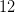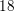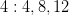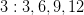# SSAT Upper Level Math : How to find the lowest / least common denominator

## Example Questions

← Previous 1

### Example Question #1 : How To Find The Lowest / Least Common Denominator

Find the least common denominator for the fractions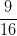and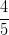.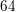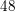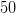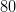Explanation:

To find the least common denominator, list out the multiples for each denominator then choose the lowest one that appears in both sets.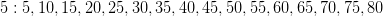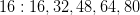### Example Question #2 : How To Find The Lowest / Least Common Denominator

Find the least common denominator for the fractions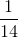and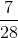.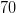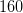Explanation:

To find the least common denominator, list out the multiples for each denominator then choose the lowest one that appears in both sets.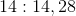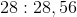### Example Question #3 : How To Find The Lowest / Least Common Denominator

What is the least common denominator for the fractionsand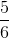?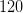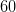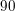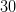Explanation:

To find the least common denominator, list out the multiples for each denominator then choose the lowest one that is in both sets.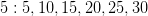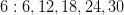### Example Question #4 : How To Find The Lowest / Least Common Denominator

Find the least common denominator for the fractionsand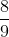.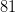Explanation:

To find the least common denominator, list out the multiples for each denominator then choose the lowest one that appears in both sets.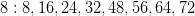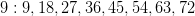### Example Question #5 : How To Find The Lowest / Least Common Denominator

Find the least common denominator for the fractions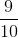and.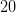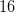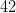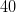Explanation:

To find the least common denominator, list out the multiples for each denominator then choose the lowest one that appears in both sets.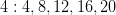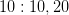### Example Question #6 : How To Find The Lowest / Least Common Denominator

Find the least common denominator for the fractions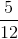and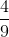.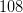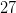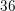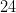Explanation:

To find the least common denominator, list out the multiples for each denominator then choose the lowest one that appears in both sets.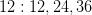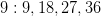### Example Question #7 : How To Find The Lowest / Least Common Denominator

Find the least common denominator for the fractionsand.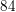Explanation:

To find the least common denominator, list out the multiples for each denominator then choose the lowest one that appears in both sets.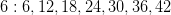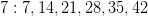### Example Question #8 : How To Find The Lowest / Least Common Denominator

Find the least common denominator for the fractions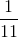and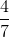.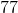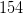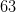Explanation:

To find the least common denominator, list out the multiples for each denominator then choose the lowest one that appears in both sets.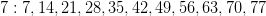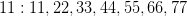### Example Question #9 : How To Find The Lowest / Least Common Denominator

Find the least common denominator for the fractionsand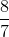.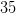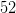Explanation:

To find the least common denominator, list out the multiples for each denominator then choose the lowest one that appears in both sets.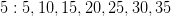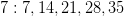### Example Question #10 : How To Find The Lowest / Least Common Denominator

Find the least common denominator for the fractions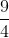and.